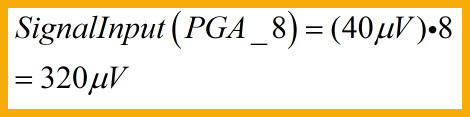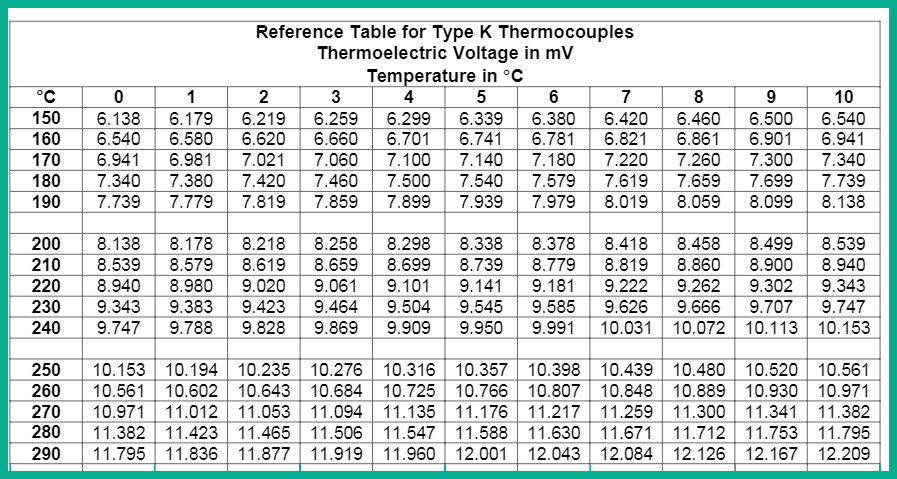Search

# Using the MCP3421 Small Signal AD Converter for Precise Small Signal Measurements

Updated: Apr 1, 2021

The MCP3421 is a single channel low-noise, high accuracy ΔΣ A/D converter with differential inputs and up to 18 bits of resolution in a small SOT-23-6 package. The on-board precision 2.048V reference voltage enables an input range of ±2.048V deferentially (Δ voltage = 4.096V). The device uses a I2C serial interface and operates from a single 2.7V to 5.5V power supply. The MCP3421 device performs conversion at rates of 3.75, 15, 60, or 240 samples per second (SPS) depending on the user controllable configuration bit settings using the I2C serial interface. This device has an on-board programmable gain amplifier (PGA). The gain can be set for x1, x2, x4, or x8 before the analog-to-digital conversion takes place. This allows the MCP3421 device to convert a smaller input signal with high resolution. The device has two conversion modes: (a) Continuous mode and (b) One-Shot mode. In One-Shot mode, the device enters a low current standby mode automatically after one conversion. This reduces current consumption during idle periods.

### Typical Applications

• Portable Instrumentation

• Weigh Scales

• Temperature Sensing with RTD, Thermistor, and Thermocouple

• Bridge Sensing for Pressure, Strain, and Force.

We will look at configuring the MCP3421 for a Thermocouple Measurement,however this applies to all types of sensor signal measuring with the device.Block Diagram from the Datasheet

The MCP3421 is ideal for small signal measurements such as the very low signals from Thermocouples and we look here at some specific's at reading a Thermocouple as shown in the Micochip Datasheet for the MCP3421 and how to use it with Flowcode Programming.

### Thermocouple Measuring (Small Signal)

The diagram below shows and example of temperature measurement using a thermocouple sensor and the MCP9800 temperature sensor. The MCP9800 is a high accuracy temperature sensor that can detect the temperature in the range of -55°C to 125°C with 1°C

accuracy.

The type K thermocouple sensor senses the temperature at the hot junction (THJ) with respect to the cold junction temperature (reference, TCJ). The temperature difference between the hot and cold junctions is represented by the voltage V1. This voltage

is then converted to digital codes by the MCP3421. In the circuit, the MCP9800 is used for cold junction compensation. The MCU computes the difference of the hot and cold junction temperatures, which is proportional to the hot junction temperature (THJ).Type K thermocouple, can measure temperature from 0°C to 1250°C degrees. The full

scale output range of the Type K thermocouple is about 50 mV. This provides 40 μV/°C

(= 50 mV/1250°C) of measurement resolution.

The detectable input signal level with 18-bit Converter Resolution is 15,625µV/PGA which

for a PGA setting of x8 = 1.953µV. The MCP3421 With this configuration, it can detect a input signal level as low as approximately 2 μV.The PGA boosts the input signal level eight times. The 40 μV/°C input from the thermocouple is amplified internally to 320 μV/°C before the conversion takes place. This results in 20.48 LSB/°C output codes. This means there are about 20 LSB output codes (or about 4.32 bits) per 1°C of change in temperature.We can calculate the number of output codes our conversion result will contain with the following Equation.(Where 50mV is the Full scale input signal,this will be your application input signal FS, if you are using it for an different application)How did we get to the number of 15.625 μV used in our calculations ,from the datasheet the following equation gives us the LSB value for each bit resolution we are using.N = Programmable bit resolution 12,14,16 or 18-bits

This shows that we can measure small signals with good accuracy with a MCP3421 ADC.

In general application using the PGA the Device the output code is found by using the

following equation.Table 1 Shows the various Maximum and Minimum Code values for each bit resolution and can be calculated as shown by the below Bit resolution.Table 1

Bit resolution is given as shown belowBit Resolution

### Flowcode Implementation.

There is an MCP3421 Component available for Flowcode which supports the various PGA and bit resolutions which the user can use to measure a application input signal.MCP3421 Component

The component supports the following Macro calls.

• Initialize - Initialize the I2C Communication

• Reset - Reset the MCP3421 to default configurations

• SampleChannelRaw - Samples the ADC Channel with user Parameters. Gain,Mode,Resolution and the Start-bit For Single Conversion / Return bit-value.

• SampleChannelVolt - Sample the ADC Channel with user Parameters /Return Voltage Value

• SampleChannel_18Bit - Samples the ADC Channel in 18-bit mode with user parameters /Return bit-Value.

• SampleChannelVolt_18bitVolt - Sample the ADC Channel with user Parameters / Return Voltage ValueThe following steps are necessary to get the temperature value

• Initialize the Component

• Reset the MCP3421

• In the Main Loop Sample the Channel and get the voltage value

• Read the Cold junction Temperature for example the MCP9800(not shown)

• Calculate the Hot junction Temperature from the 2 know values - use known methods as found in technical documents covering the actual Temperature calculation.### Temperature Calculation.

It is important to note that our example is in relation capturing these small signal such as from a thermocouple ,any user who needs to use the component for temperature measurement should inform himself regarding the Direct Calculation and also about using polynomials, a look-up table method to improve accuracy as Cold junction compensation.

The method used has a direct influence on the accuracy of the end calculated Temperature This is beyond the scope of this short article of capturing the small voltages.

We do an example of the NIST Tables to calculate the Temperature

### Simple Example of Calculating the Temperature (in our Flowcode Project)

If we are using standard NIST thermocouple reference tables or equations, the voltage VTC is a function of temperature relative to a reference temperature of 0° C. By assuming linearity and using the equation below, and assume that the voltage-versus-temperature curves with a reference temperature of 0° C are identical to curves with a reference temperature of Tref. Therefore, we can convert the measured voltage into a temperature.Using the NIST reference tables. This temperature is the difference between temperatures TTC and Tref. This is a simplified compensation method.

1. Measure the reference-junction temperature,Tref

2. Convert the measured voltage, VMEAS, into a temperature using the voltage-to- temperature relationship of the thermocouple. This temperature is approximately the difference between the thermocouple and the cold junction reference, TTC - Tref.

3. Add the temperature of the reference junction, Tref, to this value. This is the thermocouple temperature.

4. We make use of the fact that thermocouple output voltages are approximately linear over small deviations in temperature.Equation Thermocouple TemperatureExample NIST Table

We measure with our ADC 65.032mV ( Remember the measured Voltage is x8 )

and we measure with our temperature sensor MCP9800 25°C Ambient or Cold junction temperature ,We have a lookup table with the voltage for the ambient temperature in our case temperature of 25°C Ambient(cold junction translate to 1.000mV check this in the NIST tables).We have to convert the cold junction temperature to the K type Voltage

We calculate the Temperature from the ADC conversion by getting the Voltage of the Thermocouple as below.Now we add the Ambient Temperature Voltage to the Thermocouple Temperature VoltageUsing a lookup table we then find the temperature corresponding to the 9.129mV

from the NIST table you will see this is 225.5 °C approximately as we lay between 9,101 and 9.141mV.

As mentioned before there are other methods such as using Polynomials which is more complex and need more processing power. this method with the lookup tables is very light on processor resources processing power and for most applications sufficient.

Temperature Data logged a with a K-Element in an experiment using a the above techniques as described with Flowcode Codding.Temperature Logged Data

### Small Current Measuring is Another Application Example

All the above calculation methods shown is also valid and can be used in a current sense application and the Flowcode Component can be used for the programming .### Measuring Bigger input signals.

From the Datasheet:

The input voltage at each input pin must be less than the following absolute maximum input voltage limits:

• Input voltage < VDD+0.3V

• Input voltage > VSS-0.3V

Any input voltage outside this range can turn on the input ESD protection diodes, and result in input leakage current, causing conversion errors, or permanently damage the device.

This if you need to measure bigger signal use a precision voltage divider at the input pins and then sample the signal/ADC

In this example it measure a Battery Voltage it also points out the fact that the device measure differential signal it can also be used for Single ended application by grounding the VIN- pin,(Note use 1% or better Resistor for the Voltage divider circuit.### Conclusion.

The MCP3421 is a excellent ADC for small signal conversion and it is a versatile device with high accuracy and resolution.

It is an easy device to work with and it is fully supported by a Flowcode Component that help to use the device with ease.

Reference Table for Type K Thermocouples
.